# C178 – NX-19 Gas Supercompressibility

## Description

This calculates the supercompressibility factor at both line and base conditions of the input gas and uses this and the other input properties to calculate the volume correction factor and the line density of the gas.

Manual for the Determination of Supercompressibility Factors for Natural Gas- AGA PAR Research Project NX-19 (1962)

Kelton calculation reference C178

FLOCALC calculation reference F051

## Options

### Method

• Method 1: The specific gravity method
• Method 2:The analysis method
• Method 3: The methane-gravity method
• Method 4:The heating value method

Appropriate option should be chosen with regards to the input parameters the user has available to them.

## Constants

### Table 5

Gas Critical pressure (psi) Critical temperature (°R) Ideal specific gravity Ideal gas total heating value (BTU/Sft3)
Methane 673 344 0.554 994
Ethane 712 550 1.038 1742
Propane 617 666 1.523 2479
iso-Butane 529 734 2.006 3203
n-Butane 551 766 2.006 3213
iso-Pentane 483 830 2.49 3939
n-Pentane 485 846 2.49 3948
n-Hexane 440 911 2.973 4684
n-Heptane 400 972 3.46 5420
n-Octane 361 1025 3.943 6153
Carbon Dioxide 1072 510 1.519 0
Nitrogen 492 216 0.967 0
Air 547 239 1 0
Oxygen 731 278 1.105 0
Helium 33 10 0.138 0
Hydrogen 188 60 0.07 319
Hydrogen Sulphide 1306 678 1.176 630

## Calculation

### Supercompressibility and compressibility factors

The supercompressibility is calculated by: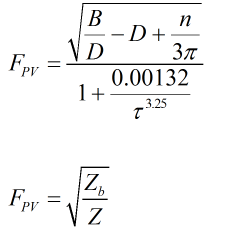Where Z = Line supercompressibility Zb = Base supercompressibility

The formulae for the compressibility factors are not explicitly given by NX-19 but are approximated using the above formulae. At pressures up to 5000 psi and temperatures up to 100°F gas compressibility factors should correlate to within 0.2% of AGA 8. The compressibility factors for line and base conditions are calculated by: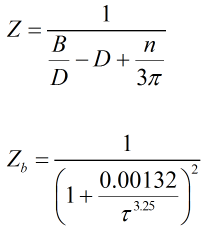Where τ = Variable based on the adjusted temperature π = Variable based on the adjusted pressure B, D, n = Are variables based on τ and π

### Temperature and pressure adjusting factors

When using the specific gravity method the temperature and pressure adjusting factors are calculated by: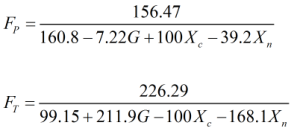When using the analysis method the temperature and pressure adjusting factors are calculated by: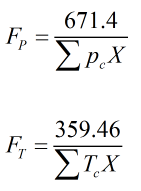When using the methane-gravity method the temperature and pressure adjusting factors are calculated by: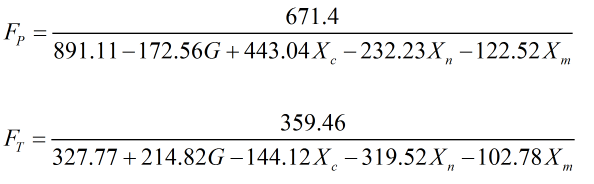When using the heating value method the temperature and pressure adjusting factors are calculated by: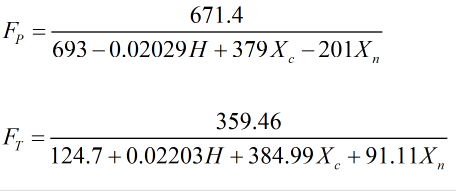Where G = Specific gravity of the gas Xc = Mole fraction of carbon dioxide Xn = Mole fraction of nitrogen Xm = Mole fraction of methane Tc = Critical temperature from Table 5 pc = Critical pressure from Table 5 H = Heating value (wet basis)

Back